# Effect of Shift in Supply on Market Equilibrium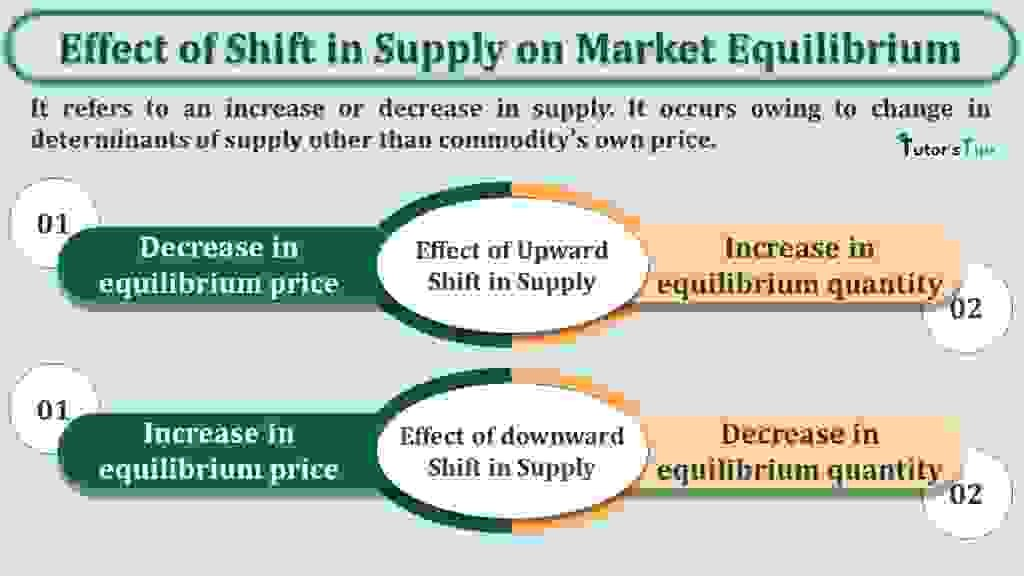The effect of Shift in supply represents the impact of an increase or decrease in the quantity supplied on the market equilibrium.

The Content covered in this article:

## The shift in the Supply Curve:

It refers to an increase or decrease in supply. It occurs owing to change in determinants of supply other than the commodity’s own price.

For example, the supply increases when there is an improvement in technology. Likewise, the supply declines when technology becomes obsolete resulting in the high cost of production.

Thus, due to change in any determinants of supply of a commodity other than its own price, the supply curve shifts from its original or initial place. The shift in supply include:

1. Upward Shift
2. Downward Shift

### Effect of Upward Shift in Supply :

The effect of an increase in supply results in a decrease in equilibrium price and an increase in equilibrium quantity.

Suppose, When the price of a commodity is Rs.75, the equilibrium quantity is initially 10 units where demand and supply are equal. Also, point A represents the initial equilibrium point. And, if the technology has improved and the supply increases in the market. As a result, the supply curve shifts from S1 to S2. Consequently, the producers’ supply for commodity increases to 20 units in the market which results in shifting from the quantity supplied from point A to B.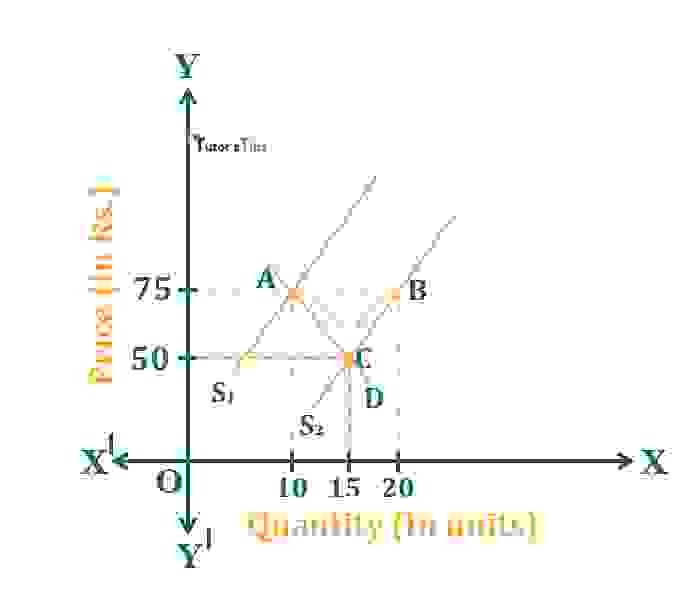Effect of increase in supply

As an immediate effect of the increase in supply, there would be excess supply in the market i.e. AB. Owing to the pressure of supply, the price of the commodity tends to decrease. Thus, it results in a fall in price to Rs.50. As price increases, the supply contracts as per the law of supply. Thus, the quantity supplied decreases to 15 units from 20 units. Also, as the price decreases, the demand also tends to increase, it occurs from 10 units to 15 units.

Thus, the process of contraction of supply and extension of demand continues till the excess supply is fully eliminated and the market clears itself.

Therefore, here,

C point is the new equilibrium point where demand and supply are equal. and, Corresponding to this, the equilibrium quantity is 15 units and the equilibrium price is Rs.50.

hence, the net effect of an increase in supply is:

1. A decrease in equilibrium price
2. Increase in equilibrium quantity.

### Effect of downward Shift in Supply:

The effect of a decrease in supply results in an increase in equilibrium price and a decrease in equilibrium quantity.

Suppose, When the price of a commodity is Rs.50, the equilibrium quantity is initially 20 units where demand and supply are equal. Also, point A represents the initial equilibrium point. And, if the technology becomes obsolete resulting in a high cost of production, the supply declines in the market. As a result, the supply curve shifts from S1 to S2. Consequently, the producers’ supply for a commodity decreases to 10 units in the market which results in shifting from quantity supplied from point A to B.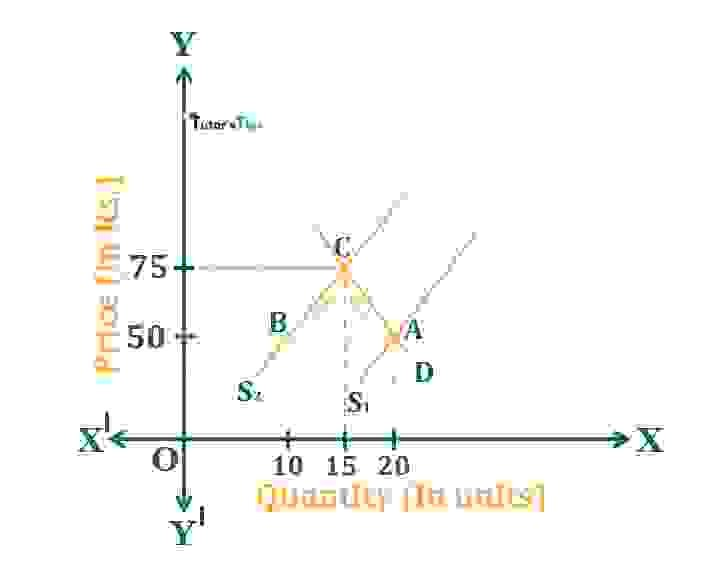Effect of decrease in supply

As an immediate effect of the decrease in supply, there would be excess demand in the market i.e. AB. Owing to the pressure of demand, the price of the commodity tends to increase. Thus, it results in a rise in price to Rs.75. As price increases, the supply extends as per the law of supply. Thus, the quantity supplied increases to 15 units from 10 units. Also, as the price increases, the demand also tends to contracts, it occurs from 20 units to 15 units.

Thus, the process of contraction of demand and extension of supply continues till the excess demand is fully eliminated and the market clears itself.

Therefore, here,

C point is the new equilibrium point where demand and supply are equal. and, Corresponding to this, the equilibrium quantity is 15 units and the equilibrium price is Rs.75.

hence, the net effect of a decrease in demand is:

1. An increase in equilibrium price
2. A decrease in equilibrium quantity.

### Some exceptional situations:

There are some exceptional cases when an increase or decrease in supply affects the market equilibrium. It shows the effect of the shift in supply

1. When demand for the commodity is perfectly elastic
2. In case, the demand for the commodity is perfectly inelastic

#### When demand for the commodity is perfectly elastic:

If the demand for a commodity is perfectly elastic, the increase or decrease in the supply of a commodity doesn’t cause any change in price. In this case, only the equilibrium quantity gets affected. Here, The effect of a shift in supply can only be seen on the equilibrium quantity.

##### When the supply of the commodity is increased:

In case, the supply of a commodity is increased with elastic demand, the equilibrium quantity increases.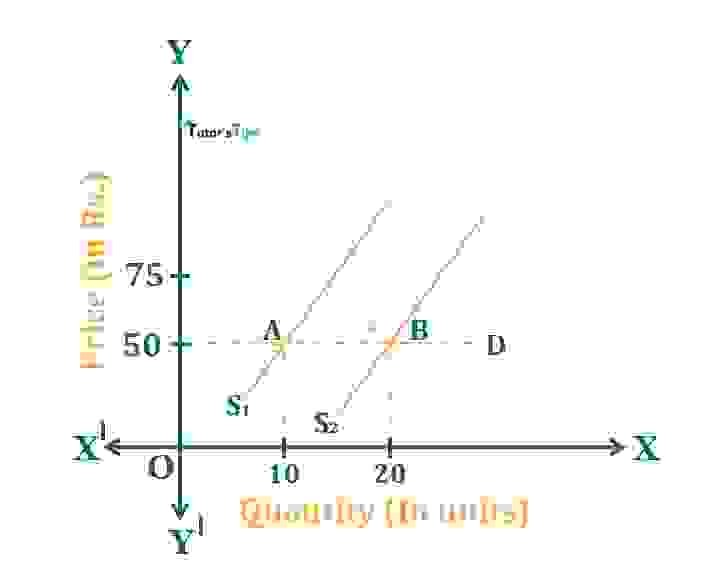Effect of increase in supply when demand is elastic

Suppose, at the initial price of Rs.50, the equilibrium quantity is 10 units where demand and supply are equal. A is the point showing the initial equilibrium point. When the supply increases in the market due to any reason, the supply curve shifts to the right, from S1 to S2. As a result, the equilibrium quantity increases to 20 units. However, there is no change in the price of the commodity. Here, the elastic demand is shown by a horizontal straight line. It means the consumers are ready to buy any quantity at any price. Also, when the supply increases in the market at a constant price, the demand also increases to 20 units. Resulting in new equilibrium point B and quantity as 20 units.

##### When the supply of the commodity is decreased:

In case, the supply of a commodity is decreased with elastic demand, the equilibrium quantity decreases.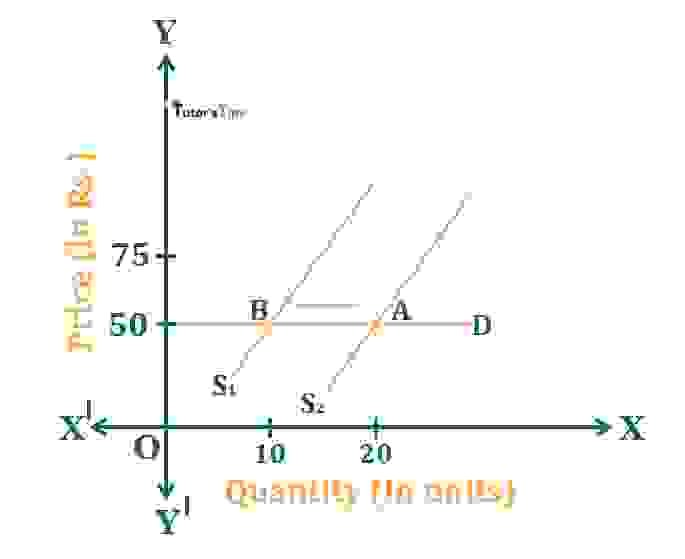Effect of a decrease in supply when demand is elastic

Suppose, at the initial price of Rs.50, the equilibrium quantity is 20 units where demand and supply are equal. A is the point showing the initial equilibrium point. When the supply decreases in the market due to any reason, the supply curve shifts to the left, from S1 to S2. As a result, the equilibrium quantity decreases to 10 units. However, there is no change in the price of the commodity. Here, the elastic demand is shown by a horizontal straight line. It means the consumers are ready to buy any quantity at any price. Also, when the supply decreases in the market at a constant price, the demand also decreases to 10 units. Resulting in new equilibrium point B and quantity as 10 units.

#### When demand for the commodity is perfectly inelastic:

If the demand for a commodity is perfectly inelastic, the increase or decrease in the supply of a commodity doesn’t cause any change in quantity. In this case, only the equilibrium price gets affected. Here, The effect of a shift in supply can only be seen on the equilibrium price.

##### When the supply of the commodity is increased:

In case, the supply of a commodity is increased with inelastic demand, the equilibrium price decreases.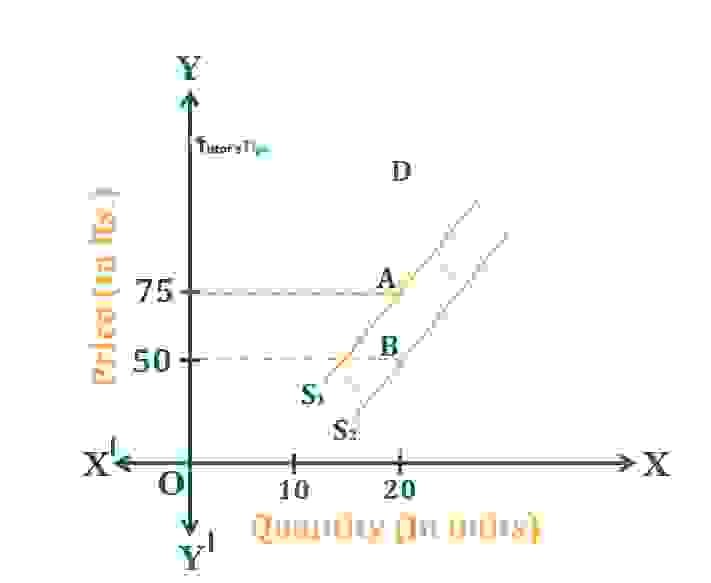Effect of increase in supply when demand is inelastic

Suppose, at the initial price of Rs.75, the equilibrium quantity is 20 units where demand and supply are equal. A is the point showing the initial equilibrium point. When the supply increases in the market due to any reason, the supply curve shifts to the right, from S1 to S2. As a result, the equilibrium price declines Rs.50. However, there is no change in the quantity demanded or supplied of the commodity. Here, the inelastic demand is shown by a vertical straight line. It means demand is constant whatever be the price of the commodity. Also, when the supply increases in the market with no change in quantity, the price decreases to Rs.50. Resulting in new equilibrium point B and price as Rs.50.

##### When the supply of the commodity is decreased:

In case, the supply of a commodity is decreased with inelastic demand, the equilibrium price increases.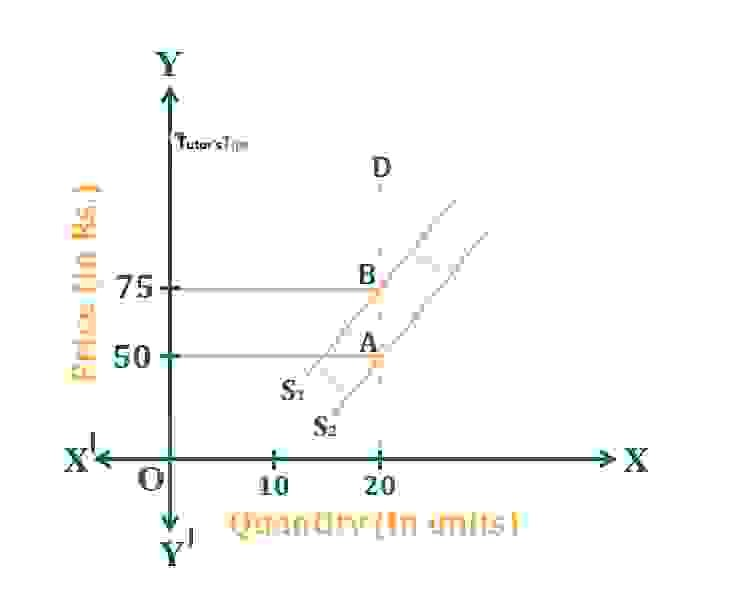Effect of a decrease in supply when demand is inelastic

Suppose, at the initial price of Rs.50, the equilibrium quantity is 20 units where demand and supply are equal. A is the point showing the initial equilibrium point. When the supply decreases in the market due to any reason, the supply curve shifts to the left, from S1 to S2. As a result, the equilibrium price increases to Rs.75. However, there is no change in the quantity demanded or supplied of the commodity. Here, the inelastic demand is shown by a vertical straight line. It means demand is constant whatever be the price of the commodity. Also, when the supply increases in the market with no change in quantity, the price increases to Rs.75. Resulting in new equilibrium point B and price as Rs.75.

Thanks!!!

Please share with your friends

Comment if you have any question

References:

Introductory Microeconomics – Class 11 – CBSE (2020-21)